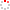#CodeStepByStep## divide

Language/Type: C++ recursion backtracking
Author: Julie Zelenski (on 2019/05/11)

Write a recursive function named `divide` that uses backtracking to divide the letters from word w into two words s1 and s2. Each letter from w must be used exactly once either in s1 or s2, and the relative order of the letters must be preserved, meaning that s1 and s2 must both be subsequences of w.

For example, the word `friendly` can be divided into `find + rely`. This solution is valid because s1 and s2 together contain all of the letters from w, the letters in s1 and s2 appear in the same relative order as they did in w, and both s1 and s2 are valid words in the English dictionary.

For some words, no valid division is possible. For example, consider dividing the word `stream`. It would not be valid to divide into `star + me` (relative order of letters not preserved), nor `stem + ram` (reuses `'m'`), nor `stem + a` (`'r'` not used), nor `seam + tr` (not in the dictionary).

You will be passed two parameters: a reference to a `Lexicon` of dictionary words, and the string w to divide. Use the `Lexicon` passed to ensure that the divided words are both found in the dictionary. If the function finds a valid division, it prints the two words and searches no further. If no valid division is found, the function prints nothing.

The following table lists some example calls to your function and possible console output, given a Lexicon called `dict`. Rows showing blank output indicate calls that were unable to find any successful division of the given word.

`Lexicon dict;   ...`
Call Possible Console Output
`divide(dict, "friendly")` `find + rely`
`divide(dict, "standing")` `sting + and`
`divide(dict, "midterm")` `mid + term`
`divide(dict, "recuperate")` `cure + repeat`
`divide(dict, "stream")`
`divide(dict, "atomic")`
`divide(dict, "czar")`
`divide(dict, "xyz")`

If there are multiple valid divisions for word w, you may print any of them. Regardless of which division you print, your code must stop after finding a single solution; do not find/print all possible solutions.

Efficiency: While your code is not required to have any particular Big-Oh, you should avoid code that is extremely inefficient or repeatedly re-explores the same path multiple times. In particular, your recursive exploration should not continue exploring a path where the prefix of the word you are examining cannot possibly make a real English word. Use the `Lexicon` to help you prune such unwanted searches. Pass all data structures by reference to avoid making copies.

Constraints: For full credit, obey the following restrictions. A solution that disobeys them can get partial credit.

• Do not declare any global variables.
• Your code can contain loops if needed to solve the problem, but your overall algorithm must be recursive and must use backtracking techniques to generate the results.
• You are allowed to define other "helper" functions if you like; they are subject to these same constraints.
Type your C++ solution code here:

This is a function exercise. Write a C++ function as described. Do not write a complete program; just the function(s) above.